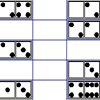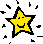#### You may also like### Days and Dates

Investigate how you can work out what day of the week your birthday will be on next year, and the year after...### Prime Magic

Place the numbers 1, 2, 3,..., 9 one on each square of a 3 by 3 grid so that all the rows and columns add up to a prime number. How many different solutions can you find?### Domino Magic Rectangle

An ordinary set of dominoes can be laid out as a 7 by 4 magic rectangle in which all the spots in all the columns add to 24, while those in the rows add to 42. Try it! Now try the magic square...

# Is it Magic or Is it Maths?

##### Age 11 to 14Challenge LevelHere are three 'tricks' to amaze your friends.

But the really clever trick is explaining to them why these 'tricks' are maths and not magic. Like all good magicians, you should practise by trying them. Can you explain how they work?This trick will impress even your maths teacher.
• Think of a number.
• Double it.
• Add $10$.
• Halve it.
• Take away you original number.
• Is your answer $5$?

Try this with a different starting number. Did you get a different result? Why does this happen?
Write the answer on a piece of paper without letting anybody see it and seal it in an envelope. Have somebody hold the envelope and at the end ask them to open it and reveal the number you wrote at the beginning. Wow, Magic!Guess how much money people have in their pockets!

Without giving you any information, ask a friend to count the value of some coins and write the amount on a piece of paper. Then ask your friend to:

• Double the amount.
• Add the first odd prime number to the new total.
• Multiply the result by 1/4 of 20.
• Subtract the lowest common multiple of 2 and 3.

For the grand finale, you ask for the final answer. Take off the last digit and you will be able to work out how much the coins are worth!Amaze your audience by working out not only their age but also what size shoe they wear! Wow them even more by telling them how the maths works. Give them the following directions but tell them not to show you any calculations:
• Write down your age.
• Multiply it by 1/5 of 100.
• Add on today's date (e.g. 2 if it's the 2nd of the month).
• Multiply by 20% of 25.
• Now add on your shoe size (if it's a half size round to a whole number).
• Finally subtract 5 times today's date.
• Show me you final answer!

Look at the answer, the hundreds are the age and the remaining digits are the shoe size. If for instance somebody shows you 1105, there are 11 hundreds - the age, and the remaining digits 05 (or 5) show the shoe size.
Now, how on earth does that work?